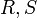# Maximal Sylow intersection

This article defines a subgroup property: a property that can be evaluated to true/false given a group and a subgroup thereof, invariant under subgroup equivalence. View a complete list of subgroup properties[SHOW MORE]

## Definition

Let$G$ be a finite group and$H$ be a subgroup of$G$. Let$p$ be a prime number. We say that$H$ is a maximal$p$-Sylow intersection in$G$ if the following two conditions hold:

• There exist two distinct$p$-Sylow subgroups$P, Q$ of$G$ such that$H = P \cap Q$.
• For any two distinct$p$-Sylow subgroups$R, S$ of$G$, the order of$R \cap S$ is not more than the order of$H$.